# Ceramic Capacitors FAQQWhat is the reason that the nominal capacitance value cannot be obtained?

A
Sometimes, when the capacitance of a high dielectric constant type ceramic capacitor is measured using an LCR meter, it is not possible to obtain the nominal capacitance value. The capacitance of a high dielectric constant type ceramic capacitor varies depending upon the temperature, voltage (AC, DC), frequency, and the passage of time, so in order to obtain the nominal capacitance value, it is necessary to measure the capacitance under the measurement conditions stipulated in sub-section 4.7 of JIS C 5101-1, 1998 "Electrostatic Capacitance," which are set out in Table 1 below.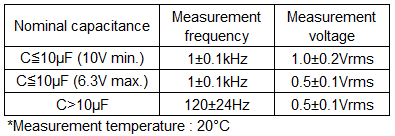Table 1

A ceramic capacitor has features such as compactness, low impedance, absence of polarity, and so on. On the other hand, it has shortcomings, in that its capacitance varies with temperature, voltage (DC, AC), frequency, and the passage of time.
The graphs below show the various characteristics that affect the measured value of the capacitance, using as examples 3216 mm size, 10uF capacitors with B characteristics and F characteristics, respectively.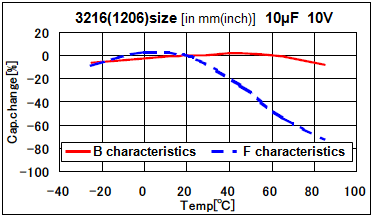Fig. 1. Temperature characteristics * Capacitance changes with temperature.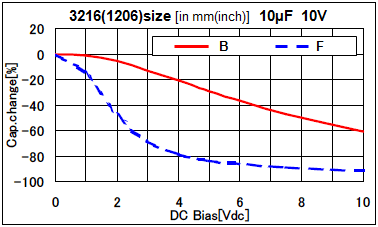Fig. 2. DC bias characteristics * Capacitance changes with DC bias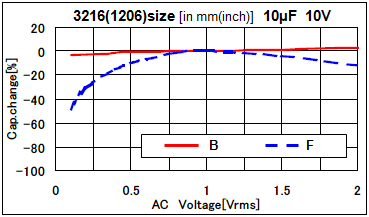Fig. 3. AC voltage characteristics * Capacitance changes with AC voltage.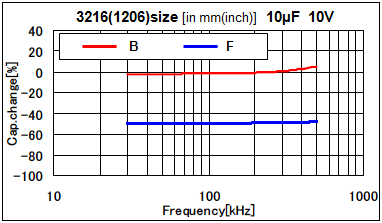Fig. 4. Frequency characteristics * Capacitance changes with frequency band.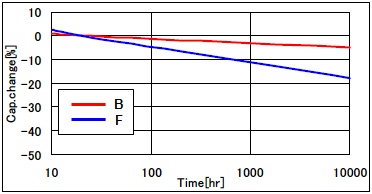Fig. 5. Aging characteristics * Capacitance change over time

As mentioned above, the capacitance of a high dielectric constant type ceramic capacitor changes depending upon the temperature, voltage (DC, AC), frequency, and the passage of time. When measuring the capacitance of a capacitor, it is necessary to have the measurement carried out under the conditions covered by the above stipulations. Also, when designing the circuit, adequately take into account the characteristics of the ceramic capacitor under the conditions of the working environment.

Bias characteristics, temperature characteristics, frequency characteristics, etc. can be confirmed with this software. (SimSurfing)
SimSurfing
How to use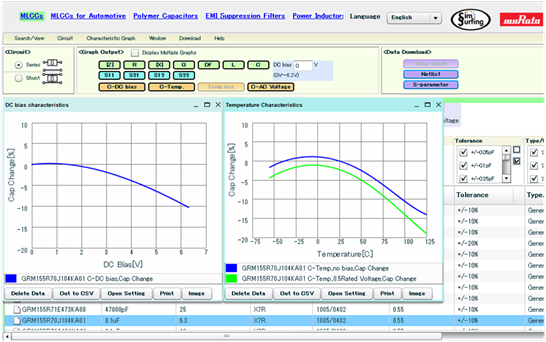#### Related FAQ

We appreciate your cooperation with the FAQ improvement questionnaire.